# User:Tohline/Appendix/Ramblings/RiemannB74C692

(Difference between revisions)
Jump to: navigation, search
 Revision as of 20:39, 30 May 2020 (view source)Tohline (Talk | contribs) (→Quantitative Animation)← Older edit Revision as of 20:42, 30 May 2020 (view source)Tohline (Talk | contribs) (→Quantitative Animation)Newer edit → Line 129: Line 129: - We have chosen to specify the time-varying angle in increments of 60° and to end the animation sequence after the ellipsoid (or, alternatively, the laboratory frame) has completed exactly five full spins.  As a result — ignoring any zero-point offsets — one of the ANGLE arrays will vary from 0° to 5 × 360° = 1800° in 60° intervals (if spinning counter-clock-wise) or will vary from 1800° to 0° in -60° intervals.  The number of discrete data values that must be specified for each of the three arrays is therefore, by choice, N = (1800/60)+1 = 31. + We have chosen to specify the time-varying angle in increments of 60° and to end the animation sequence after the ellipsoid (or, alternatively, the laboratory frame) has completed exactly five full spins.  As a result — ignoring any zero-point offsets — one of the ANGLE arrays will vary from 0° to 5 × 360° = 1800° in 60° intervals (if spinning counter-clock-wise) or will vary from 1800° to 0° in -60° intervals (if spinning clock-wise).  Again ignoring any zero-point offset, all the data values in the other ANGLE array will be 0°.  The number of discrete data values that must be specified for each of the three arrays is therefore, by choice, N = (1800/60)+1 = 31. ==COLLADA Model Files== ==COLLADA Model Files==

# Another S-type Example b74c692

This chapter is an extension of the chapter we have titled, "Riemann Meets COLLADA & Oculus Rift S." In that chapter we used as our first example of a Riemann S-type ellipsoid the model with parameters, (b/a, c/a) = (0.41, 0.385). Here we construct a model with parameters, (b/a, c/a) = (0.74, 0.692). Other closely related chapters are listed below under the heading, "See Also".

 |   Tiled Menu   |   Tables of Content   |  Banner Video   |  Tohline Home Page   |

## Key Physical Parameters

The model that we have chosen to use in our second successful construction of a COLLADA-based, 3D and interactive animation has the following properties; this model has been selected from Table 2 of our accompanying discussion of Riemann S-type ellipsoids: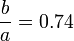$~\frac{b}{a} = 0.74$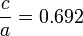$~\frac{c}{a} = 0.692$ Direct Adjoint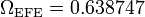$~\Omega_\mathrm{EFE} = 0.638747$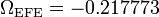$~\Omega_\mathrm{EFE} = - 0.217773$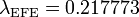$~\lambda_\mathrm{EFE} = 0.217773$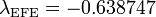$~\lambda_\mathrm{EFE} = - 0.638747$

The subscript "EFE" on Ω and λ means that the relevant frequency is given in units that have been adopted in [EFE], that is, in units of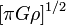$~[\pi G\rho]^{1 / 2}$. In Figure 1a, the solid purple circular marker (where the pair of purple lines cross) identifies the location of this model in the "c/a versus b/a" diagram that appears as Figure 2 on p. 902 of S. Chandrasekhar (1965); essentially the same diagram appears in §49 (p. 147) of [EFE].

## Coding Steps

Here we begin with a working model of b41c385 and use incremental changes in the COLLADA-based code to construct a working model of b74c692.

• Pulling from the earlier modeling subsection titled, Final Touches
• Final17.dae   TERRIFIC  !:
Both hands of the clock now continue to cycle smoothly (through 9.126 "hours" = 9h 8m) while the laboratory frame completes 5.0 full spin periods — which will be 5.0 full spins of the ellipsoid as viewed from the inertial frame. This works in both visualization venues.
• Inertial17.dae:
This is identical to "Final17.dae" except that the system is viewed from the inertial frame of reference. This works in both visualization venues.
• [KEEP] Inertial21.dae:
Here, we set the "nudging" angle of the MidPlane to 0°, but moved the "nudging" angle of the ellipsoid from 0° to just 1°. This appears to work in both visualization venues.
• Pulling from a different earlier subsection titled, Multiple Lagrangian Fluid Elements
• MultiLagrange23.dae --> TestMulti23.dae:
This model shows the motion of nine (small, red cube) Lagrange particles whose motion is confined to the equatorial plane of the Riemann ellipsoid. This nicely illustrates that the volume occupied by a region of fluid remains unchanged as the fluid moves around the ellipsoid.   This works in both visualization venues!
• [KEEP] MultiLagrange26.dae:
Here, we set the "nudging" angle of the MidPlane to 1°, and moved the "nudging" angle of the ellipsoid from 5° to 0°. Also, we bumped the purple's opacity up from 0.6 to 0.8. This appears to work in both visualization venues.
• TestMulti52.dae
Same as MultiLagrange23.dae --> TestMulti23.dae, except the starting ellipsoid angle is nudged by 45° instead of 5°.
• [KEEP] TestMulti56.dae:
Same as MultiLagrange26.dae, except we have attempted to replace the alpha-numeric labeling. SUCCESS! Works in all three visualization venues: Mac "Preview", PC "3D Viewer", and Oculus RIft S.
• Facebook glTF Transmutator (v1.5)
I am only now appreciating that, when a ".glb"-formatted file is imported into the Oculus Rift S inventory, the importation step is handled by Facebook's glTF Transmutator (v1.5).
• TestMulti70b.dae
Same as TestMulti56.dae, except we have inserted the correct EllipsoidOctant geometries (drawn from b74c692 Old_DRot.dae). This works in both visualization venues.

## Quantitative Animation

### First_rotation_euler_Z

Whether the observer is viewing the system from an inertial frame of reference or a rotating frame of reference, this animation section specifies the rate at which the ellipsoid spins and the rate at which the "laboratory" is spinning. Three sets of data must be specified:

1. The "First_rotation_euler_Z-input-array", which specifies TIME.
2. The "First_rotation_euler_Z-output-array", which specifies how the ANGLE of orientation of the ellipsoid varies with TIME.
3. The "Frame_rotation_euler_Z-output-array", which specifies how the spin ANGLE of the laboratory frame varies with TIME.

We have chosen to specify the time-varying angle in increments of 60° and to end the animation sequence after the ellipsoid (or, alternatively, the laboratory frame) has completed exactly five full spins. As a result — ignoring any zero-point offsets — one of the ANGLE arrays will vary from 0° to 5 × 360° = 1800° in 60° intervals (if spinning counter-clock-wise) or will vary from 1800° to 0° in -60° intervals (if spinning clock-wise). Again ignoring any zero-point offset, all the data values in the other ANGLE array will be 0°. The number of discrete data values that must be specified for each of the three arrays is therefore, by choice, N = (1800/60)+1 = 31.

## COLLADA Model Files

b74c692DI.dae [Direct Inertial Frame]    …     a COLLADA code containing nnnn lines of <xml>-formatted ASCII text

<?xml version="1.0" encoding="UTF-8" standalone="no" ?>


b74c692DRot.dae [Direct Rotating Frame]    …     a COLLADA code containing nnnn lines of <xml>-formatted ASCII text

<?xml version="1.0" encoding="UTF-8" standalone="no" ?>


b74c692AI.dae [Adjunct Inertial Frame]    …     a COLLADA code containing nnnn lines of <xml>-formatted ASCII text

<?xml version="1.0" encoding="UTF-8" standalone="no" ?>


b74c692ARot.dae [Adjunct Rotating Frame]    …     a COLLADA code containing nnnn lines of <xml>-formatted ASCII text

<?xml version="1.0" encoding="UTF-8" standalone="no" ?>


# See Also

 © 2014 - 2021 by Joel E. Tohline |   H_Book Home   |   YouTube   | Appendices: | Equations | Variables | References | Ramblings | Images | myphys.lsu | ADS | Recommended citation:   Tohline, Joel E. (2021), The Structure, Stability, & Dynamics of Self-Gravitating Fluids, a (MediaWiki-based) Vistrails.org publication, https://www.vistrails.org/index.php/User:Tohline/citation